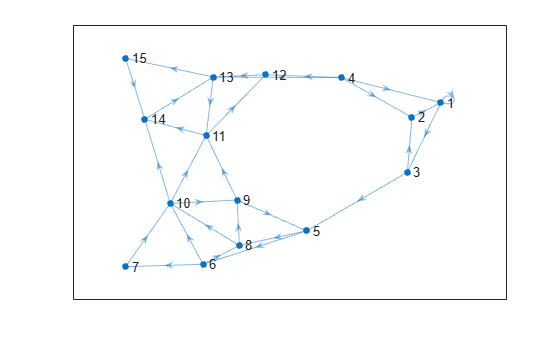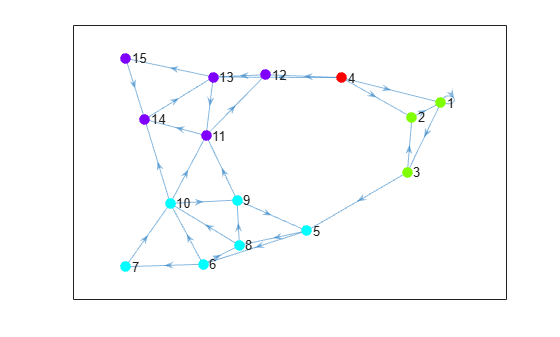Documentation

### This is machine translation

Mouseover text to see original. Click the button below to return to the English version of the page.

Note: This page has been translated by MathWorks. Click here to see
To view all translated materials including this page, select Country from the country navigator on the bottom of this page.

# condensation

Graph condensation

## Syntax

``C = condensation(G)``

## Description

example

````C = condensation(G)` returns a directed graph `C` whose nodes represent the strongly connected components in `G`. This reduction provides a simplified view of the connectivity between components.```

## Examples

collapse all

Create and plot a graph that contains several strongly connected components. Highlight the strongly connected components.

```s = [1 1 2 3 3 4 4 4 4 5 5 6 6 6 7 8 8 9 9 10 10 10 11 11 12 13 13 14 15]; t = [1 3 1 2 5 1 2 12 13 6 8 7 8 10 10 9 10 5 11 9 11 14 12 14 13 11 15 13 14]; G = digraph(s,t); p = plot(G);``````bins = conncomp(G); p.MarkerSize = 7; p.NodeCData = bins; colormap(hsv(4))```Use `condensation` to represent each component as a single node. Color the nodes based on the components they represent.

```C = condensation(G); p2 = plot(C); p2.MarkerSize = 7; p2.NodeCData = 1:4; colormap(hsv(4))```## Input Arguments

collapse all

Input graph, specified as a `digraph` object. Use `digraph` to create a directed graph object.

Example: `G = digraph([1 2],[2 3])`

## Output Arguments

collapse all

Condensation graph, returned as a `digraph` object. `C` is a directed acyclic graph (DAG), and is topologically sorted. The node numbers in `C` correspond to the bin numbers returned by `conncomp`.

`condensation` determines the nodes and edges in `C` by the components and connectivity in `G`:

• `C` contains a node for each strongly connected component in `G`.

• `C` contains an edge between node `I` and node `J` if there is an edge from any node in component `I` to any node in component `J` of `G`.

Download ebook